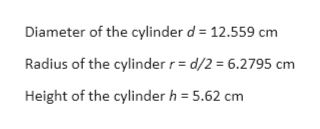# The outside dimensions of a cylindrical soda can are reported as 12.559 cm for the diameter and 5.62 cm for the height. (a) How many significant figures will the total outside area have: (1) two, (2) three, (3) four, or (4) five? Why? (b) What is the total outside surface area of the can in square meters?

Question
6 views

The outside dimensions of a cylindrical soda can are reported as 12.559 cm for the diameter and 5.62 cm for the height. (a) How many significant figures will the total outside area have: (1) two, (2) three, (3) four, or (4) five? Why? (b) What is the total outside surface area of the can in square meters?

check_circle

Step 1

Given:help_outlineImage TranscriptioncloseDiameter of the cylinder d 12.559 cm Radius of the cylinder r = d/2 = 6.2795 cm Height of the cylinder h 5.62 cm fullscreen
Step 2

(a) As the height of the cylinder has least number of significant digits i.e. three

So final answer will also contain three significant digits.

Option 2 is correct.

Step 3

(b) To calculate total outsid...

### Want to see the full answer?

See Solution

#### Want to see this answer and more?

Solutions are written by subject experts who are available 24/7. Questions are typically answered within 1 hour.*

See Solution
*Response times may vary by subject and question.
Tagged in

### Units and Dimensions